JEE  >  Short & Long Answer Question: Laws of Motion- 2

# Short & Long Answer Question: Laws of Motion- 2 Notes | Study Additional Documents and Tests for JEE - JEE

## Document Description: Short & Long Answer Question: Laws of Motion- 2 for JEE 2022 is part of Documents for Additional Documents and Tests for JEE preparation. The notes and questions for Short & Long Answer Question: Laws of Motion- 2 have been prepared according to the JEE exam syllabus. Information about Short & Long Answer Question: Laws of Motion- 2 covers topics like and Short & Long Answer Question: Laws of Motion- 2 Example, for JEE 2022 Exam. Find important definitions, questions, notes, meanings, examples, exercises and tests below for Short & Long Answer Question: Laws of Motion- 2.

Introduction of Short & Long Answer Question: Laws of Motion- 2 in English is available as part of our Additional Documents and Tests for JEE for JEE & Short & Long Answer Question: Laws of Motion- 2 in Hindi for Additional Documents and Tests for JEE course. Download more important topics related with Documents, notes, lectures and mock test series for JEE Exam by signing up for free. JEE: Short & Long Answer Question: Laws of Motion- 2 Notes | Study Additional Documents and Tests for JEE - JEE
 1 Crore+ students have signed up on EduRev. Have you?

Q.51 Sand is thrown on tracks covered with snow. Why?
When tracks are covered with snow, there is considerable reduction of frictional force. So, the driving is not safe. When sand is thrown on the snow-covered tracks, the frictional force increases. So, safe driving is possible.
Q.52 Why are wheels of automobiles made circular?

Answer: Circular wheels roll on the road and rolling friction comes into play during the motion of automobile. Rolling friction is less than the sliding friction. It is due to this reason that wheels of automobiles are made circular.
Q.53 Proper inflation of tyres of vehicles saves fuel. Why?
When the tyre is properly inflated, the area of contact between the tyre and the ground is reduced. This reduces rolling friction. Consequently, the auto- mobile covers greater distance for the same quantity of fuel consumed.
Q.54 It is difficult to move a cycle along a road with its brakes on. Explain?
: When the cycle is moved with brakes on, the wheels can only skid. So, the friction is sliding in nature. Since the sliding friction is greater than rolling friction, therefore, it is difficult to move a cycle with its brakes on.
Q.55 A large size brake on bicycle is as effective as small one. Comment.

Answer: Action of brakes is based upon friction. But the friction is independent of the area of surfaces in contact so long as the normal reaction remains the same. Hence, large size brakes and normal size trakes will be equally effective if the material of brakes remains unchanged.
Q.56 Why is it difficult to put a cycle into motion than to maintain its motion?
To put a cycle into motion, one needs to overcome limiting friction while to maintain its motion, one needs to overcome kinetic friction. Limiting friction is greater than the kinetic friction. So it is difficult to put a cycle into motion than to maintain its motion.
Q.57 How does friction help us in walking?
Due to friction, we are able to push the ground backward during walking. The reaction of the ground helps us to move forward.
Q.58 Why do we slip on a rainy day?
On a rainy day, the wet ground becomes very smooth. The friction between our feet and the ground is greatly reduced. It causes us to slip.
Q.59 Why is it difficult to walk on sand?
Because of its yielding nature, sand cannot exert as much forward thrust as hard ground does.
Q.60 Why fictional force gets increased when a surface is polished beyond a certain limit?

Answer: When surfaces are highly polished, the area of contact between them increases. As a result of this, a large number of atoms and molecules lying on both the surfaces start exerting strong attractive forces on each other and therefore frictional force increases.
Q.61 When a person walks on a rough surface, the frictional force exerted by the surface on the person is opposite to direction of his motion. State whether it true or false?

Answer: False. When a person walks, he pushes the ground backward with his foot. The tendency of the foot when it is in the contact of the earth is to move backward. Hence the force of friction acts in the forward direction, i.e., in a direction in which the man walks.
Q.62 Why has a horse to pull a cart harder during the first few steps of his motion? Or A horse has to apply more force to start a cart than to keep it moving. Why?

Answer: During the first few steps of his motion, the horse has to work against the limiting friction and once the cart starts moving, the horse has to work against kinetic friction which is less than limiting friction.
Q.63 Is it unreasonable to expect the coefficient of friction to exceed unity?

Answer: No. The coefficient is less than unity for normal plane surfaces. But when the surfaces are so irregular that they have sharp minute projections and cavities on them, then the coefficient of friction may exceed unity.
Q.64 Is friction a non-conservative force?

Answer: Yes. When the direction of motion of a body reverses, the direction of friction is also reversed. Work has 1 to be done against friction both during forward and return journey i.e., work done against friction along a closed path is not zero. So friction is a non-conservative force.
Q.65 For uniform circular motion, does the direction of centripetal force depend on the sense of rotation?

Answer: No. Whether a body revolves clockwise or anticlockwise, the centripetal force always acts along the radius towards the centre of the circle.
Q.66 A stone tied at the end of a string is whirled in a circle. When the string breaks, the stoneflies away tangentially? Explain why.
The instantaneous velocity of the stone moving round the circle is along the tangent to the circular path. When the string breaks, the centripetal force vanishes. Due to the inertia of motion, the stone flies away tangentially.
Q.67 Why does a child in a merry-go-round press the side of his seat radially outward?

Answer: In accordance with Newton's third law, the seat will press the child inward, providing the necessary centripetal force.
Q.68 Why does skidding takes place generally on a rainy day along a curved path?
The force of friction between the road and the tyres of the vehicle may not be sufficient to provide the necessary centripetal force.
Q.69 A car is taking a sudden turn to the left. A passenger in the front seat finds himself sliding towards the door. Explain, indicating the forces acting on the passenger and the car at this instant.
The passenger in the front seat slides towards the door i.e., away from the circular turn. This is because of the centrifugal force acting on the passenger.
Q.70 The outer rail of a curved railway track is generally raised over the inner. Why?
When the outer rail of a curved railway track is raised over the inner, the horizontal component of the normal reaction of the rails provides the necessary centripetal force for the train to enable it move along the curved path.
Q.71 A motor cyclist is going in a vertical circle. What is the necessary condition so that he may not fall down?

Answer: The necessary condition that the motor cyclist may not fall down is mυ2/r≥ mg i.e., υ≥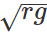at the highest point and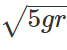at the lowest point.
Q.72 A bucket containing water is rotated in a vertical circle. Explain, why the water does not fall.
Answer: For its revolution in a vertical circle, water [n the bucket needs a centripetal force. The weight of the water due to which water can fall is used up in providing the necessary centripetal force and the water does not fall.
Q.73 Why does a pilot looping a vertical loop lot fall down even at the highest point?

Answer: At the highest point of the vertical loop, the weight of the pilot due to which he can fall is used up in providing him the necessary centripetal force.
Q.74 What is the maximum possible velocity at the lowest position for oscillation of a simple pendulum of length L. What can happen to the motion if the velocity exceeds this value?

Answer: The maximum possible velocity at the lowest point for oscillation of simple pendulum =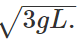When velocity exceeds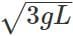but is less than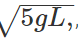the bob leaves the vertical circle. When υ=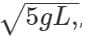the bob completes the vertical circle.
Q.75 A cricket player lowers his hands to catch the ball safely. Explain, why?
The impulse is equal to the product of the force exerted by the ball and the time of catch. By lowering the hands, the time of catch increases. Then the force exerted on the hands becomes much smaller and it does not hurt the cricketer.
Q.76  It is easy to catch a table tennis ball than a cricket ball even both are moving with same velocity. Why?
Due to its small mass, the momentum of the table tennis ball is much smaller than that of the cricket ball of same velocity. Less force is required to stop the table tennis ball than the cricket ball. Hence it is easy to catch the table tennis ball than the cricket ball.
Q.77  Why does a cyclist bend inwards while riding along a curved road?

Answer: A cyclist bends inwards because then the horizontal component of the normal reaction of the ground provides the necessary centripetal force for going along the curved road.
Q.78 The motion of a particle of mass m is described by y=ut+1/2gt2  Find the force acting on the particle.

Answer: We have y=ut+1/2gt2 Velocity,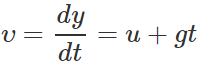Acceleration,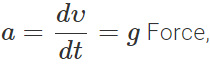F=ma=mg Thus the given equation describes the motion of a particle under acceleration due to gravity and y is the position coordinate in the direction of g.
Q.79 A particle of mass 0.3 kg is subjected to a force of F=−kx with k=15Nm−1. What will be its initial acceleration, if it is released from a point 20 cm away from the origin?
Here k=15Nm−1, x=20cm=0.20m,m=0.3kg F=−kx=15×0.20=−3N Acceleration,     a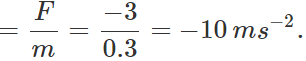Q.80  block of mass M is pulled along a horizontal frictionless surface by a rope of mass m. A force P is applied at the free end of the rope. Find the force exerted by the rope on the block.
Answer: If a is the acceleration produced, then P=(M+m)a   or a=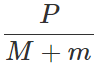Force exerted by the rope on the block F=Ma=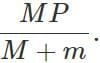Q.81 Three forces F1,Fand F3 are acting on a particle of mass m, such that F2 and F3 are mutually perpendicular and under their effect, the particle remains stationary. What will be the acceleration of the particle, if the force F1 is removed?
Answer: As the particle remains stationary under the action of the three forces, so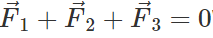When force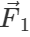is removed, the net force left is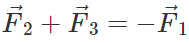Acceleration, a=−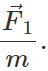Q.82 A spring balance is attached to the ceiling of a lift. A man hangs his bag on the spring and the spring reads 49 N, when the lift is stationary. What will be the reading of the spring balance, if the lift moves downward with an acceleration of 5ms−2.

Answer: When the lift is stationary. The reaction of the spring is equal to weight of the bag. ∴  R=mg=49N or m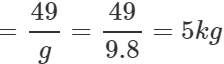When the lift moves downward. The reaction is R′=m(g−a)=5(9.8−5)=24N.
Q.83 Why does Newton's first law of motion appear to be contradicted in our day-to-day life?

Answer: In our day-to-day observations, we find that when we push a block lying on the surface of a table, it covers some distance before it stops. At first sight, it seems to contradict Newton's first law of motion. However, the motion of the block is being opposed by the force of friction between the block and the table and also by air resistance. In the absence of such opposing forces, the block would continue to move on its own.
Q.84 Explain, why (a) The passengers are thrown forward from their seats, when a speeding bus stops suddenly. (b) Does a cricketer move his hand backwards while holding a catch? (c) Is the boat pushed away when a man jumps out of the boat?

Answer: (a) This is due to inertia of motion. When the speeding bus stops suddenly, lower part of the body in contact with the seat stops. The upper part of the body of the passengers tends to maintain its uniform motion. Hence the passengers are thrown forward.

(b) When the ball is caught, the impulse received by the hands is equal to the product of the force exerted by the ball and the time taken to complete the catch. By moving the hands backwards, the cricketer increases the time of catch. The force exerted on his hands becomes much smaller and it does not hurt him.

(c) Initially, the total momentum of the boat and the man is zero. As the man jumps out of the boat, he gains momentum in the forward direction. To conserve momentum, the boat also gains an equal and opposite momentum. So the boat moves away from the shore.
Q.85 Explain: (a) Why are ball bearings used in machinery? (b) Why does a horse have to apply more force to start a cart than to keep it moving? (c) What is the need for banking the tracks?

Answer: (a) By using ball bearings between the moving parts of a machinery, the sliding friction gets converted into rolling friction. The rolling friction is much smaller than sliding friction. This reduces power dissipation.

(b) During the first few steps of his motion, the horse has to work against the limiting friction and once the cart starts moving, the horse has to work against kinetic friction which is less than limiting friction.

(c) When the circular track is banked, the horizontal component of the normal reaction of the road provides the necessary centripetal force for the vehicle to move it along the curved path. This reduces wear and tear of the tyres.
Q.86 The speed of driving a car safely depends upon the range of headlight. Explain.

Answer: By the range of the headlight of a car, we mean the maximum distance(s) up to which an obstacle on the road can be seen by the driver in the darkness. The driver has to stop the car before it reaches the obstacle. The retarding force acting on the car is constant. If, on applying brakes, the retardation in the car is a, then to stop the car within distance s, the speed υ of the car should be less than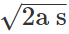. Thus the speed of the car depends on the range (s) of the headlight.
Q.87 A bird is sitting on the floor of a closed glass cage and the cage is in the hand of a girl. Will the girl experience any change in the weight of the cage when the bird (i) starts flying in the cage with a constant velocity (ii) flies upwards with acceleration (iii) flies downwards with acceleration?

Answer: In a closed cage, the inside air is bound with the cage.

(i) As the acceleration is zero, there is no change m the weight of the cage.

(ii) In this case, the reaction R is given by R−Mg=Ma or R=M(g+a) Thus the cage will appear heavier than before.

(iii) In this case, the reaction R is given by Mg−R=Ma or R=M(g−a) Thus the cage will appear lighter than before.
Q.88 A man stands in a lift going downward with uniform velocity. He experiences a loss of weight at the start but not when lift is in uniform motion. Explain why?

Answer: The apparent weight is given by R=W−ma=mg−ma or R=m(g−a) Since lift is in acceleration in the start, a≠0, so R<mg.When lift comes in uniform motion, acceleration ceases (a=0) and man experiences his own weight.
Q.89 Aeroplanes having wings fly at low altitudes while jet planes fly at high altitudes. Why?
The wings of the aeroplane push the external air backward and the aeroplane moves forward by the reaction of the pushed air. At lower altitude the air is dense and so the aeroplane receives sufficient reactional force to move forward. In the jet plane the external air is sucked into the plane and compressed. Hence for the air to be dense is not only unnecessary but also undesirable. The reason is that jet planes fly with very large velocity. If air is dense, then due to the air-friction the plane will become very hot. Therefore, jet planes fly at high altitude where air-density is very small.
Q.90 A person (mass m) is hanging from a rope fastened to a stationary balloon (mass M). If the person climbs along the rope, then with what velocity the balloon will move and in what direction? The velocity of the person relative to the rope is υ.

Answer: The initial momentum of the person and balloon is zero. Hence when the person climbs, the balloon will come down with such a velocity υ that the total momentum be still zero. The velocity of the person dative to earth is (υ−u). Hence
m(υ−u)−Mu=0 or u=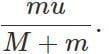Q.91 A long rope is hanging, passing over a pulley. Two monkeys of equal weights climb up from the opposite ends of the rope. One of them climbs up more rapidly relative to rope. Which monkey will reach first at the top? The pulley is frictionless and the rope is massless and inextensible.
There is no external force which may provide momentum to any monkey. The monkeys themselves give equal momenta to each other (through the rope). Therefore, two monkeys will climb up the rope at the same rate relative to the earth. As their masses are equal, they will reach the top simultaneously.
Q.92 When a ball is thrown upward, its momentum first increases and then decreases. Is the conservation of momentum violated in this process?
: No. The combined momentum of the ball and the earth is conserved. The ball attracts the earth by the same force as the earth attracts the ball. When the ball moves upward, its momentum decreases in the upward direction but simultaneously the momentum of the earth increases in the upward direction at the same rate. Similarly, when the ball falls down, its momentum increases in the downward direction but simultaneously the momentum of the earth increases in the upward direction at the same rate.
Q.93 A disc of mass m is placed on a table. A stiff spring is attached to it and is vertical. To the other end of the spring is attached a disc of negligible mass.  What minimum force should be applied to the upper disc to press the spring such that the lower disc is lifted off the table when the external force is suddenly removed?

Answer: The minimum applied force should be mg. When a force mg is applied vertically downwards on the upper disc, the lower disc gets pressed against the floor with a force equal to mg. The floors exerts an upward reaction equal to mg. When the external force is suddenly removed, this force of reaction lifts up the lower disc.
Q.94 Can a single isolated force exist in nature? Give reason.

Answer: No. According to Newton's third law of motion, to every action there is always an equal and opposite reaction. So the forces always exist in pairs. When we talk of a single force, we are just considering only one aspect of the mutual interaction.
Q.95 A body is dropped from the ceiling of a transparent cabin falling freely towards the earth. Describe the motion of the body as observed by an observer (i) sitting in the cabin (ii) standing on the earth.

Answer: (i) The body will appear stationary in air. (ii) The body will appear falling freely under gravity.
Q.96 A ball is suspended by a cord from the ceiling of a motor car. What will be the effect on the position of the ball if (i) the car is moving with uniform velocity (ii) the car is moving with accelerated motion and (iii) the car is turning towards right?
(i)The ball will remain suspended vertically. (ii) The ball will move in backward direction. (iii) The ball will move towards left.
Q.97 When brakes are applied in a bicycle, the brake shoes apply frictional force on the wheels. The forces are internal for the system (bicycle). For retardation, external forces must act on the system. The force of friction at the road surface remains the same before and after the brakes are applied(f=μR). How does the bicycle stop?
When brakes are applied, the wheels are prevented from rolling. During rolling, the friction at the road surface does not cause retardation. When rolling is prevented, the wheels have to slip on the road. The friction of the road now causes retardation.
Q.98 A light string passing over a smooth pulley connects two blocks of masses m1 and m2 (vertically). If the acceleration of the system is g/8,find the ratio of the two masses.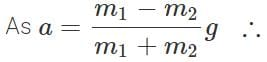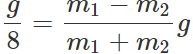or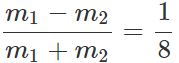or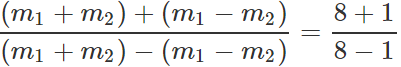or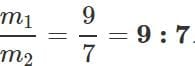The document Short & Long Answer Question: Laws of Motion- 2 Notes | Study Additional Documents and Tests for JEE - JEE is a part of the JEE Course Additional Documents and Tests for JEE.
All you need of JEE at this link: JEE

## Additional Documents and Tests for JEE

11 videos|159 docs|14 tests
 Use Code STAYHOME200 and get INR 200 additional OFF

## Additional Documents and Tests for JEE

11 videos|159 docs|14 tests

Track your progress, build streaks, highlight & save important lessons and more!

,

,

,

,

,

,

,

,

,

,

,

,

,

,

,

,

,

,

,

,

,

;